Q-Chem 5.0 User’s Manual

# 10.16 Linear–Scaling Computation of Electric Properties

The search for new optical devices is a major field of materials sciences. Here, polarizabilities and hyperpolarizabilities provide particularly important information on molecular systems. The response of the molecular systems in the presence of an external monochromatic oscillatory electric field is determined by the solution of the TDSCF equations, where the perturbation is represented as the interaction of the molecule with a single Fourier component within the dipole approximation: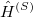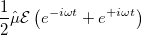(10.66)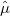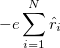(10.67)

Here,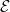is the E-field vector,the corresponding frequency,the electronic charge and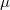the dipole moment operator. Starting from Frenkel’s variational principle the TDSCF equations can be derived by standard techniques of perturbation theory . As a solution we yield the first- [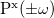and second-order [e.g.,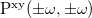] perturbed density matrices with which the following properties are calculated:

• Static polarizability: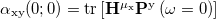• Dynamic polarizability: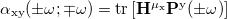• Static hyperpolarizability: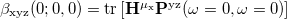• Second harmonic generation: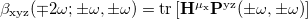• Electro-optical Pockels effect: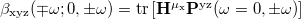• Optical rectification: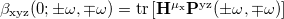where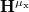is the matrix representation of thecomponent of the dipole moments.

The TDSCF calculation is the most time consuming step and scales asymptotically as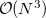because of the AO/MO transformations. The scaling behavior of the two-electron integral formations, which dominate over a wide range because of a larger pre-factor, can be reduced by LinK/CFMM from quadratic to linear (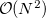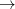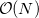).

Third-order properties can be calculated with the equations above after a second-order TDSCF calculation (MOPROP: 101/102) or by use of Wigner’s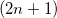rule  (MOPROP: 103/104). Since the second order TDSCF depends on the first-order results, the convergence of the algorithm may be problematically. So we recommend the use of 103/104 for the calculation of first hyperpolarizabilities.

These optical properties can be computed for the first time using linear-scaling methods (LinK/CFMM) for all integral contractions . Although the present implementation available in Q-Chem still uses MO-based time-dependent SCF (TDSCF) equations both at the HF and DFT level, the pre-factor of this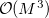scaling step is rather small, so that the reduction of the scaling achieved for the integral contractions is most important. Here, all derivatives are computed analytically.

Further specifications of the dynamic properties are done in the section $fdpfreq in the following format: $fdpfreq
property
frequencies
units
$end  The first line is only required for third order properties to specify the kind of first hyperpolarizability: • StaticHyper Static Hyperpolarizability • SHG Second harmonic generation • EOPockels Electro-optical Pockels effect • OptRect Optical rectification Line number 2 contains floating-point values for the frequencies of the perturbations. Alternatively, for dynamic polarizabilities an equidistant sequence of frequencies can be specified by the keyword WALK (see example below). The last line specifies the units of the given frequencies: • au Frequency (atomic units) • eV Frequency (eV) • nm Wavelength (nm) • Hz Frequency (Hertz) • cmInv Wavenumber (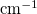) ## 10.16.1 Examples for Section$fdpfreq

Example 10.255  Static and Dynamic polarizabilities, atomic units:

$fdpfreq 0.0 0.03 0.05 au$end


Example 10.256  Series of dynamic polarizabilities, starting with 0.00 incremented by 0.01 up to 0.10:

$fdpfreq walk 0.00 0.10 0.01 au$end


Example 10.257  Static first hyperpolarizability, second harmonic generation and electro-optical Pockels effect, wavelength in nm:

$fdpfreq StaticHyper SHG EOPockels 1064 nm$end


## 10.16.2 Features of Mopropman

• Restricted/unrestricted HF and KS-DFT CPSCF/TDSCF

• LinK/CFMM support to evaluate Coulomb- and exchange-like matrices

• DIIS acceleration

• Support of LSDA/GGA/Hybrid XC functionals listed below

• Analytical derivatives

The following XC functionals are supported:

Exchange:

• Dirac

• Becke 88

Correlation:

• Wigner

• VWN (both RPA and No. 5 parameterizations)

• Perdew-Zunger 81

• Perdew 86 (both PZ81 and VWN (No. 5) kernel)

• LYP

## 10.16.3 Job Control

The following options can be used:

MOPROP
 Specifies the job for mopropman.

TYPE:
 INTEGER

DEFAULT:
 0 Do not run mopropman.

OPTIONS:
 1 NMR chemical shielding tensors. 2 Static polarizability. 3 Indirect nuclear spin–spin coupling tensors. 100 Dynamic polarizability. 101 First hyperpolarizability. 102 First hyperpolarizability, reading First order results from disk. 103 First hyperpolarizability using Wigner’srule. 104 First hyperpolarizability using Wigner’srule, reading first order results from disk.

RECOMMENDATION:
 None

MOPROP_PERTNUM
 Set the number of perturbed densities that will to be treated together.

TYPE:
 INTEGER

DEFAULT:
 0

OPTIONS:
 0 All at once.Treat the perturbed densities batch-wise.

RECOMMENDATION:
 Use the default. For large systems, limiting this number may be required to avoid memory exhaustion.

MOPROP_CONV_1ST
 Sets the convergence criteria for CPSCF and 1st order TDSCF.

TYPE:
 INTEGER

DEFAULT:
 6

OPTIONS: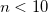Convergence threshold set to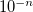.

RECOMMENDATION:
 None

MOPROP_CONV_2ND
 Sets the convergence criterion for second-order TDSCF.

TYPE:
 INTEGER

DEFAULT:
 6

OPTIONS:Convergence threshold set to.

RECOMMENDATION:
 None

MOPROP_MAXITER_1ST
 The maximum number of iterations for CPSCF and first-order TDSCF.

TYPE:
 INTEGER

DEFAULT:
 50

OPTIONS:Set maximum number of iterations to.

RECOMMENDATION:
 Use the default.

MOPROP_MAXITER_2ND
 The maximum number of iterations for second-order TDSCF.

TYPE:
 INTEGER

DEFAULT:
 50

OPTIONS:Set maximum number of iterations to.

RECOMMENDATION:
 Use the default.

MOPROP_ISSC_PRINT_REDUCED
 Specifies whether the isotope-independent reduced coupling tensor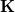should be printed in addition to the isotope-dependent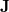-tensor when calculating indirect nuclear spin–spin couplings.

TYPE:
 LOGICAL

DEFAULT:
 FALSE

OPTIONS:
 FALSE Do not print. TRUE Print.

RECOMMENDATION:
 None

MOPROP_ISSC_SKIP_FC
 Specifies whether to skip the calculation of the Fermi contact contribution to the indirect nuclear spin–spin coupling tensor.

TYPE:
 LOGICAL

DEFAULT:
 FALSE

OPTIONS:
 FALSE Calculate Fermi contact contribution. TRUE Skip Fermi contact contribution.

RECOMMENDATION:
 None

MOPROP_ISSC_SKIP_SD
 Specifies whether to skip the calculation of the spin–dipole contribution to the indirect nuclear spin–spin coupling tensor.

TYPE:
 LOGICAL

DEFAULT:
 FALSE

OPTIONS:
 FALSE Calculate spin–dipole contribution. TRUE Skip spin–dipole contribution.

RECOMMENDATION:
 None

MOPROP_ISSC_SKIP_PSO
 Specifies whether to skip the calculation of the paramagnetic spin–orbit contribution to the indirect nuclear spin–spin coupling tensor.

TYPE:
 LOGICAL

DEFAULT:
 FALSE

OPTIONS:
 FALSE Calculate paramagnetic spin–orbit contribution. TRUE Skip paramagnetic spin–orbit contribution.

RECOMMENDATION:
 None

MOPROP_ISSC_SKIP_DSO
 Specifies whether to skip the calculation of the diamagnetic spin–orbit contribution to the indirect nuclear spin–spin coupling tensor.

TYPE:
 LOGICAL

DEFAULT:
 FALSE

OPTIONS:
 FALSE Calculate diamagnetic spin–orbit contribution. TRUE Skip diamagnetic spin–orbit contribution.

RECOMMENDATION:
 None

MOPROP_DIIS
 Controls the use of Pulay’s DIIS in solving the CPSCF equations.

TYPE:
 INTEGER

DEFAULT:
 5

OPTIONS:
 0 Turn off DIIS. 5 Turn on DIIS.

RECOMMENDATION:
 None

MOPROP_DIIS_DIM_SS
 Specified the DIIS subspace dimension.

TYPE:
 INTEGER

DEFAULT:
 20

OPTIONS:
 0 No DIIS.Use a subspace of dimension.

RECOMMENDATION:
 None

SAVE_LAST_GPX
 Save last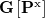when calculating dynamic polarizabilities in order to call mopropman in a second run with MOPROP = 102.

TYPE:
 INTEGER

DEFAULT:
 0

OPTIONS:
 0 False 1 True

RECOMMENDATION:
 None